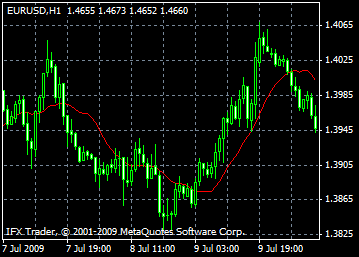# Moving average indicator formula and settings: description, adjustment and application

The Moving Average indicator (MA) shows the average price for a certain period of time. This indicator is calculated by mathematical averaging of the asset price for the given period. As the price dynamics change, its average reading either increases or declines.

There are four types of moving averages method: the Simple, or Arithmetic, Exponential, Smoothed, and Linear Weighted ones. The MA indicator can be used to calculate a consequent set of data that includes opening and closing prices, highs and lows, trade volume, or readings of other moving average indicators. Sometimes, traders use a moving average of other moving averages.

The distinguishing feature of a moving average is its weight coefficient that is assigned to the last data in a set. Thus, the Simple moving average indicator shows prices that have the same weight coefficient within the given period. The Exponential moving averages and Linear Weighted moving averages assign a bigger weight coefficient to the last prices in a set.

The moving average indicator is often interpreted as the correlation between its dynamics and the price dynamics. If the MA reading is lower than the price of a trading instrument, then it should be considered as a signal to buy. And vice versa, if the MA reading is above the price, a sell trade should be initiated.

Trading on moving averages enables investors to make decisions in accordance with the current developments in the market. In other words, they buy after prices hit a bottom and sell after quotes make highs. The MA method can also be applied to other indicators. The correlation is the same: if an indicator moves above the MA reading, it is likely to continue rising; and if it goes below the MA reading, it will dip lower.

#### Types of moving averages:

Simple Moving Average (SMA)

Exponential Moving Average (EMA)

Smoothed Moving Average (SMMA)

Linear Weighted Moving Average (LWMA)#### Formulas for calculating moving averages:

Simple Moving Average, SMA

The Simple, or Arithmetic, moving average is calculated by adding together closing prices of certain periods, 12 hours for example, and dividing the sum by the number of periods.

SMA = SUM (CLOSE, N)/N

SUM — the sum;

CLOSE (i) — the closing price of the current period;

N — the number of periods.

Exponential Moving Average, EMA

The Exponential moving average is calculated by adding a certain part of the current closing price to the previous MA reading. As for the EMA, closing prices have bigger weight coefficient. Here is the formula for calculation of the EMA:

EMA = (CLOSE (i)*P) + (EMA (i-1)*(100-P))

CLOSE (i) — the closing price of the current period;

EMA (i-1) — the MA reading of the previous period;

P — the percentage of using price readings.

Smoothed Moving Average, SMMA

The formula for calculating the first reading of the Smoothed moving average is similar to the one used for calculation of the Simple moving average.

SUM1 = SUM(CLOSE, N)

SMMA1 = SUM1/N

The second and subsequent readings are calculated in the following way:

SMMA (i) = (SUM1-SMMA1+CLOSE (i))/N

SUM — the sum;

SUM1 — the sum of closing prices of N periods that start from the previous bar;

SMMA (i - 1) — the smoothed moving average of the previous bar;

SMMA (i) — the smoothed moving average of the current bar (except the first one);

CLOSE (i) — the current closing price;

N — the period of smoothing.

Linear Weighted Moving Average, LWMA

In the Linear Weighted Moving Average the latest data in the set is assigned a bigger weight coefficient, while the earlier prices have a smaller weight. The LWMA is calculated by multiplying each of the closing prices by a certain weight coefficient.

LWMA = SUM (Close (i)*i, N)/SUM (i, N)

SUM — the sum;

CLOSE(i) — the current closing price;

SUM (i, N) — the sum of weight coefficient;

N — the period of smoothing.

Take the first step towards your goal
Open account